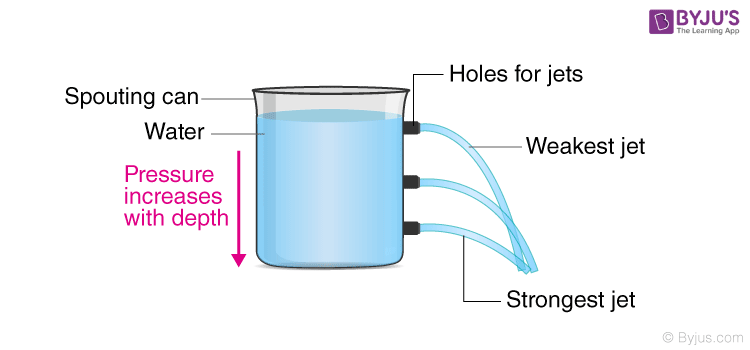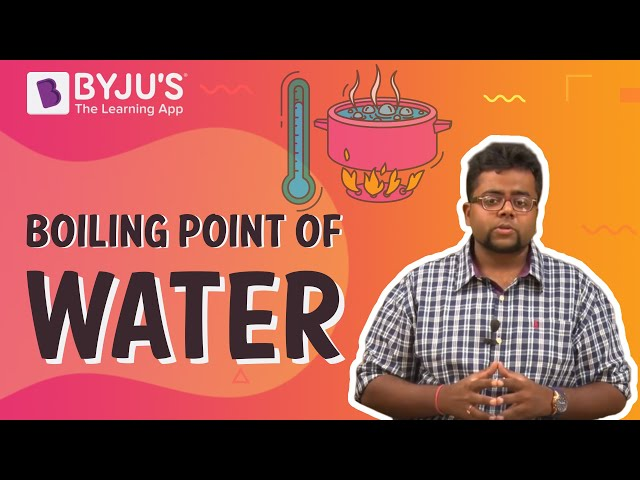Checkout JEE MAINS 2022 Question Paper Analysis : Checkout JEE MAINS 2022 Question Paper Analysis :

# Hydrostatic Pressure

We know that matter in all states exerts pressure. Liquids and gases exert equal pressure on all sides of a container. The normal force exerted by a liquid per unit area of the surface in contact is called hydrostatic pressure or pressure of the liquid. In this article, let us discuss hydrostatic pressure in detail.

## What is Hydrostatic Pressure?

Hydrostatic pressure is defined as

“The pressure exerted by a fluid at equilibrium at any point of time due to the force of gravity”.

Hydrostatic pressure is proportional to the depth measured from the surface as the weight of the fluid increases when a downward force is applied.

The fluid pressure can be caused by gravity, acceleration or forces when in a closed container. Consider a layer of water from the top of the bottle. There is a pressure exerted by the layer of water acting on the sides of the bottle. As we move down from the top of the bottle to the bottom, the pressure exerted by the top layer on the bottom adds up.  This phenomenon is responsible for more pressure at the bottom of the container.## Hydrostatic Pressure Formula

Following is the formula used to calculate the hydrostatic pressure:

 p = ρgh

where,

• p is the pressure exerted by the liquid in N.m-2, Pa
• ρ is the density of the liquid in kg.m-3, slugs.ft-3
• g is the acceleration due to gravity taken as 9.81m.s-2
• h is the height of the fluid column in m

### Hydrostatic Pressure Vs Depth of Water

Following is the table explaining how the depth of water value changes with the pressure:

 Depth of water Pressure ft m Pa bar 1 0.30 2990 0.03 5 1.52 14949.99 0.15 10 3.05 29899.98 0.30 15 4.57 44849.97 0.45

## Hydrostatic Pressure Derivation

Consider a column of water with the total volume V and A as the base surface area.

The weight of the water is:

W = mg

We know that the mass of water is just the density of water with volume X. Therefore,

W = ρVg

Volume is the product of surface area and height. Therefore,

V = Ah

W =ρAhg

Using the formula of pressure:

$$\begin{array}{l}p=\frac{F}{A}\end{array}$$
$$\begin{array}{l}p=\frac{\rho Ahg}{A}\end{array}$$

p = ρhg

### Solved Problems on Hydrostatic Pressure

Q1. What is the pressure acting on the water at a depth of 1m at 4°C?

Ans: The depth of water column = 1m

The density of water at 4°C = 1000kg/m3

The formula used is: p = ρgh

= (1000kg/m3)(9.81m.s-2)(1m)

= 9810 Pa.

Q2. What is the pressure acting on the water at a depth of 3ft at 32°F?

Ans: The depth of water column = 3ft

The density of water at 32°F = 1.940 slugs.ft-3

The formula used is: p = ρgh

= (1.940 slugs.ft-3)(32.17405ft.s-2)(3ft)

=187.3lb.ft-2.
See the video below and learn about the boiling point of water.## Frequently Asked Questions – FAQs

p = ρgh

### What is hydrostatic pressure?

The pressure exerted by a fluid at equilibrium at any point of time due to the force of gravity.

### When a downward force is applied, what happens to the weight of the fluid?

The weight of the fluid increases.

### What is fluid pressure?

The pressure at a point within a fluid arising due to the weight of the fluid is known as fluid pressure.

### How is the density of the fluid represented?

The density of the fluid is represented as ρ.

Hope you have learned about hydrostatic pressure along with its formula. Stay tuned with BYJU’S for more such interesting articles. Also, register to “BYJU’S – The Learning App” for loads of interactive, engaging Physics-related videos and an unlimited academic assist.

Test Your Knowledge On Hydrostatic Pressure!